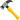# Second order partial derivatives vanish?

Gold Member
At the end of a long proof I came across something in tensor calculus that seems too good to be true. And if something seems too good to be true ...

The something is that a second order partial derivative vanishes if one of the parts in the denominator is in the same reference frame as the numerator. That is for example
\begin{align}

\frac{{\partial }^2x^{\mu }}{\partial x^{\rho }\partial x^{{\mu }^{'}}}=\frac{{\partial }^2x^{\mu }}{\partial x^{{\mu }^{'}}\partial x^{\rho }}=0 \\

\end{align}The equality of the first two parts follows because partial derivatives commute. We have \begin{align}

\frac{{\partial }^2x^{\mu }}{\partial x^{{\mu }^{'}}\partial x^{\rho }}=\frac{\partial }{\partial x^{{\mu }^{'}}}\left(\frac{\partial x^{\mu }}{\partial x^{\rho }}\right) \\

\end{align}but\begin{align}

\frac{\partial x^{\mu }}{\partial x^{\rho }}={\delta }^{\mu }_{\rho } \\

\end{align}where ##{\delta }^{\mu }_{\rho }## is the Kronecker delta which is a constant. (3) seems very reasonable because when ##\mu \neq \rho ##, ##\partial x^{\mu }## and ##\partial x^{\rho }## are orthogonal so ##{\partial x^{\mu }}/{\partial x^{\rho }}## vanishes and when ##\mu =\rho ##, ##{\partial x^{\mu }}/{\partial x^{\rho }}=1##.

So equation (1) is the derivative of a constant which always vanishes. QED.

Have I made a very stupid mistake? Or am I stating something everybody knows?

Staff Emeritus
The equality of the first two parts follows because partial derivatives commute.

This principle needs to be used with caution. For a fixed coordinate system, if ##x## and ##y## are two different coordinates, then it is definitely the case that ##\frac{\partial}{\partial x} \frac{\partial}{\partial y} f(x,y) = \frac{\partial}{\partial y} \frac{\partial}{\partial x} f(x,y)##

However, if ##x## and ##y## are from DIFFERENT coordinate systems, then that isn't necessarily true. Here's a trivial example. Consider one dimension, so there is only one coordinate, ##x##. We can switch to another coordinate system ##x' = \sqrt{x}##. Consider the function ##cos(x)##

Take the derivative with respect to ##x##. You get ##-sin(x)##.
Re-express it in terms of ##x'##. You get ##-sin((x')^2)##.
Take the derivative with respect to ##x'##. You get ##-2 x' cos((x')^2) = -2 \sqrt{x} cos(x)##.

Now do it in the opposite order.
Re-express ##cos(x)## in terms of ##x'##. You get ##cos((x')^2)##.
Take the derivative with respect to ##x'##. You get ##-2x' sin((x')^2)##.
Re-express it in terms of ##x##. You get ##-2\sqrt{x} sin(x)##.
Take the derivative with respect to ##x##. You get ##-\frac{1}{\sqrt{x}} sin(x) - 2\sqrt{x} cos(x)##.

Those aren't the same.

•WWGD and George Keeling
Gold Member
This principle needs to be used with caution.
Oh dear. I'll have to think about cautions tomorrow.

Gold Member
This principle needs to be used with caution.
Thanks for taking the trouble to work the example. It teaches me two things:
1) Apply that caution
2) Try an example when I get another 'to good to be true result'
Following rule (1) we believe$$\frac{{\partial }^2f}{\partial x^{\rho }\partial x^{{\nu }}}=\frac{{\partial }^2f}{\partial x^{{\nu }}\partial x^{\rho }}$$Following rule (2) test$$f\left(x,y\right)=x^2{\mathrm{sin} y\ }$$then $$\frac{\partial }{\partial x}\left(\frac{\partial }{\partial y}f\right)=\frac{\partial }{\partial x}\left(x^2{\mathrm{cos} y\ }\right)=2x{\mathrm{cos} y\ }$$and$$\frac{\partial }{\partial y}\left(\frac{\partial }{\partial x}f\right)=\frac{\partial }{\partial y}\left(2x{\mathrm{sin} y\ }\right)=2x{\mathrm{cos} y\ }$$That works!
My question was really when ##f=x^\mu## which is a coordinate. So I should test ##f=y## and ##f=z##. The second order derivatives both rapidly vanish. I mow need to revise my rule to:
The second order derivative of a coordinate by two other coordinates in the same reference frame vanishes. Not so impressive.I will now now have to rework my problemOnce again stevendaryl, thanks for the help you have given with with this and other problems.